Open in App
Not now

# Split dataframe in Pandas based on values in multiple columns

• Last Updated : 19 Dec, 2021

In this article, we are going to see how to divide a dataframe by various methods and based on various parameters using Python. To divide a dataframe into two or more separate dataframes based on the values present in the column we first create a data frame.

## Python3

 `# importing pandas as pd ` `import` `pandas as pd ` ` `  ` `  `# dictionary of lists ` `dict` `=` `{``'First_Name'``: [``"Aparna"``, ``"Pankaj"``, ``"Sudhir"``,  ` `                       ``"Geeku"``, ``"Anuj"``, ``"Aman"``, ` `                       ``"Madhav"``, ``"Raj"``, ``"Shruti"``], ` `        ``'Last_Name'``: [``"Pandey"``, ``"Gupta"``, ``"Mishra"``,  ` `                      ``"Chopra"``, ``"Mishra"``, ``"Verma"``,  ` `                      ``"Sen"``, ``"Roy"``, ``"Agarwal"``], ` `        ``'Email_ID'``: [``"apandey@gmail.com"``, ``"pankaj@gmail.com"``, ` `                     ``"sumishra23@gmail.com"``, ``"cgeeku@yahoo.com"``, ` `                     ``"anuj24@gmail.com"``, ``"amanver@yahoo.com"``, ` `                     ``"madhav1998@gmail.com"``, ``"rroy7@gmail.com"``, ` `                     ``"sagarwal36@gmail.com"``], ` `        ``'Degree'``: [``"MBA"``, ``"BCA"``, ``"M.Tech"``, ``"MBA"``, ``"B.Sc"``, ` `                   ``"B.Tech"``, ``"B.Tech"``, ``"MBA"``, ``"M.Tech"``], ` `        ``'Score'``: [``90``, ``40``, ``75``, ``98``, ``94``, ``90``, ``80``, ``90``, ``95``]} ` ` `  `# creating dataframe ` `df ``=` `pd.DataFrame(``dict``) ` ` `  `print``(df) `

Output: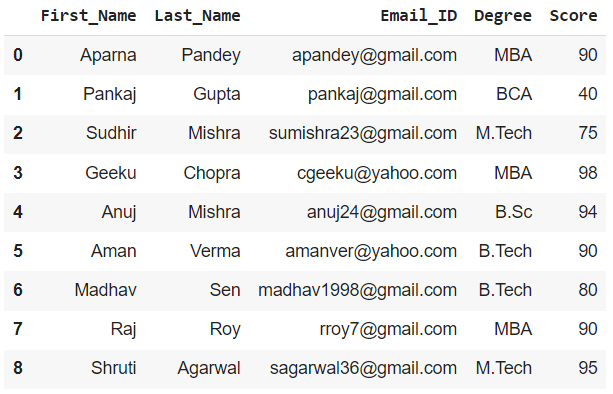## Method 1: By Boolean Indexing

We can create multiple dataframes from a given dataframe based on a certain column value by using the boolean indexing method and by mentioning the required criteria.

Example 1: Creating a dataframe for the students with Score >= 80

## Python3

 `# creating a new dataframe by applying the required  ` `# conditions in []  ` `df1 ``=` `df[df[``'Score'``] >``=` `80``] ` ` `  `print``(df1)`

Output: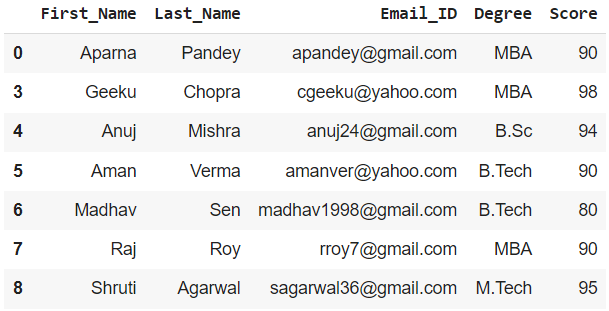Example 2: Creating a dataframe for the students with Last_Name as Mishra

## Python3

 `# Creating on the basis of Last_Name ` `dfname ``=` `df[df[``'Last_Name'``] ``=``=` `'Mishra'``] ` ` `  `print``(dfname)`

Output: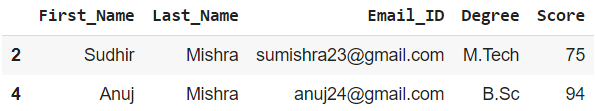We can do the same for other columns as well by putting the appropriate condition

## Method 2: Boolean Indexing with mask variable

We create a mask variable for the condition of the column in the previous method

Example 1: To get dataframe of students with Degree as MBA

## Python3

 `# creating the mask variable with appropriate ` `# condition ` `mask_var ``=` `df[``'Degree'``] ``=``=``'MBA'` ` `  `# creating a dataframe ` `df1_mask ``=` `df[mask_var] ` ` `  `print``(df1_mask)`

Output :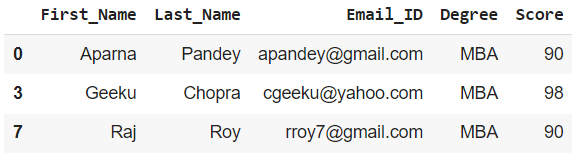Example 2: To get a dataframe for the rest of the students

To get the rest of the values in a dataframe we can simply invert the mask variable by adding a ~(tilde) after it.

## Python3

 `# creating dataframe with inverted mask variable ` `df2_mask ``=` `df[~mask_var] ` ` `  `print``(df2_mask)`

Output :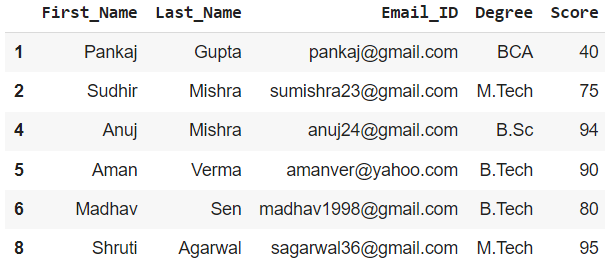## Method 3: Using groupby() function

Using groupby() we can group the rows using a specific column value and then display it as a separate dataframe.

Example 1: Group all Students according to their Degree and display as required

## Python3

 `# Creating an object using groupby ` `grouped ``=` `df.groupby(``'Degree'``) ` ` `  `# the return type of the object 'grouped' is  ` `# pandas.core.groupby.generic.DataFrameGroupBy. ` ` `  `# Creating a dataframe from the object using get_group(). ` `# dataframe of students with Degree as MBA. ` `df_grouped ``=` `grouped.get_group(``'MBA'``) ` ` `  `print``(df_grouped) `

Output: dataframe of students with Degree as MBA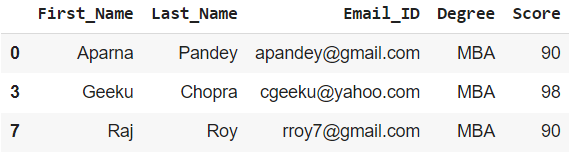Example 2: Group all Students according to their Score and display as required

## Python3

 `# Creating another object using groupby ` `grouped2 ``=` `df.groupby(``'Score'``) ` ` `  `# the return type of the object 'grouped2' is  ` `# pandas.core.groupby.generic.DataFrameGroupBy. ` ` `  `# Creating a dataframe from the object  ` `# using get_group() dataframe of students ` `# with Score = 90 ` `df_grouped2 ``=` `grouped2.get_group(``90``) ` ` `  `print``(df_grouped2)`

Output: dataframe of students with Score = 90.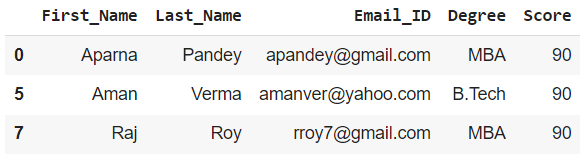My Personal Notes arrow_drop_up
Related Articles